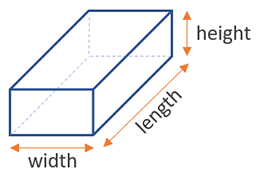# Volume of a Box Calculator

Use this box volume calculator to easily calculate the volume of a rectangular box or tank from its length, width and height (depth) in any metric: mm, cm, meters, km, inches, feet, yards, miles... Useful for shipping dimensions in cubic meters / feet.

### Calculation results

60,000 cm3 Box volume
Share calculator:

Embed this tool:
get code

## Volume of a box formula

The volume of a rectangular box can be calculated if you know its three dimensions: width, length and height. The formula is then volumebox = width x length x height. Illustration below:Measuring the sides of a rectangular box or tank is easy. The result from the calculation, using our volume of a rectangular box calculator or otherwise, will always be in the length unit used, cubed. Make sure to have all measurements in the same unit, if you are doing the math by hand, and if using our calculator - make sure to select the appropriate metric. If you measured the lengths in inches, the result will be in cubic inches. If the length was in feet, the result will be in cubic feet, and so on for yards3, miles3, mm3, cm3, meters3.

## How to calculate the volume of a box?

To find the volume of a rectangular box or tank, you need to take three measurements, then multiply them. In practical situations, you might have a plan or engineering schematic in which the measurements are all given making your task significantly easier. That is fairly easy to do in your mind if the numbers are small, but quickly gets inconvenient if the numbers become large, where a volume of a box calculator such as the above gets really useful.

It should be noted that "volume of a box" may not be a precise term, since if we take the box to be an abstract mathematical concept, its walls have zero thickness and the box itself has zero volume. However, in practical situations we usually talk about the volume in terms of the space enclosed by the box or tank, and that is what the calculator above calculates. If that is what you are calculating and want to know what it can hold, make sure to measure its internal, not external dimensions, otherwise you will need to then subtract the volume of the walls.

## Example: find the volume of a rectangular box

Three measurements need to be known to compute the volume of any box with a rectangular shape: the lengths of two sides of its base, as well as the height. For example, if the two sides of the base are of length 3 inches and 6 inches respectively, and the height of the box is 2 inches, then using the box volume equation results in 3 x 6 x 2 = 18 x 2 = 36 in3 (cubic inches).

Alternatively, the area of one side of a box might be given, and the height relative to that side. In that case just multiply the area by the height. For example, if the base of the box is 25 sq ft and the side of the box ortogonal to it is 4 feet long, then the box volume is 25 x 4 = 100 cu ft.

## Cubic Meter / Cubic Feet cargo calculations

Often one needs to calculate the volume of shipping containers, boxes, crates, tanks, and other transportation devices. Usually there will be a technical specification by the manufacturer, or at least you would know the standard based on which the container was produced, giving you minimum allowable dimensions.

If you want a more specialized calculator, which will tell you how many items if given dimensions you can fit, try our container calculator and our pallet calculator.

## Other applications

The rectangular box is one of the most widely used body shapes across science, engineering, architecture, due to its good structural properties and resistance to force from different sides. Most homes and rooms in them are rectangular boxes, most transportation containers are boxes or have a box-like shape. Calculating aquarium volume, swimming pool volume, or the volume of soil, mulch or plant nutrients you need for a given garden area are other common uses. Boxes and tanks from wood, steel, bricks, concrete, glass, and other materials are also easy to produce, compared to more complicated shapes.

Another benefit of the rectangular shape is how easily smaller shapes can fit into larger ones, without the need of complex calculations, rotations and overall arrangement. So, this box volume calculator has a lot of possible applications.

#### Cite this calculator & page

If you'd like to cite this online calculator resource and information as provided on the page, you can use the following citation:
Georgiev G.Z., "Volume of a Box Calculator", [online] Available at: https://www.gigacalculator.com/calculators/volume-of-box-calculator.php URL [Accessed Date: 01 Apr, 2023].